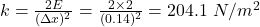## Suppose that 2 J of work is needed to stretch a spring from its natural length of 32 cm to a length of 46 cm. (a) How much work (in J) is ne

Question

Suppose that 2 J of work is needed to stretch a spring from its natural length of 32 cm to a length of 46 cm. (a) How much work (in J) is needed to stretch the spring from 37 cm to 41 cm

in progress 0
6 months 2021-07-18T23:17:55+00:00 1 Answers 7 views 0

the work required is 0.163 J

Explanation:

Given;

Energy applied to the spring, E = 2 J

initial length of the spring, x₀ = 32 cm

final length of the spring, x₁ = 46 cm

Extension of the spring, Δx = x₁ – x₀ = 46 cm – 32 cm = 14 cm = 0.14 m

The spring constant is calculated as follows;

E = ¹/₂kΔx²The extension of the spring when it is stretched from 37 cm + 41 cm:

Δx =  41 cm – 37 cm = 4 cm = 0.04 m

The work required:

W = ¹/₂kΔx²

W = ¹/₂ x (204.1) x (0.04)²

W = 0.163 J

Therefore, the work required is 0.163 J